# 移动测试开发 界面相似度比对算法实践

opentest-oper@360.cn · March 11, 2019 · Last by 笑哼 replied at April 04, 2019 · 1399 hits

## 哈希算法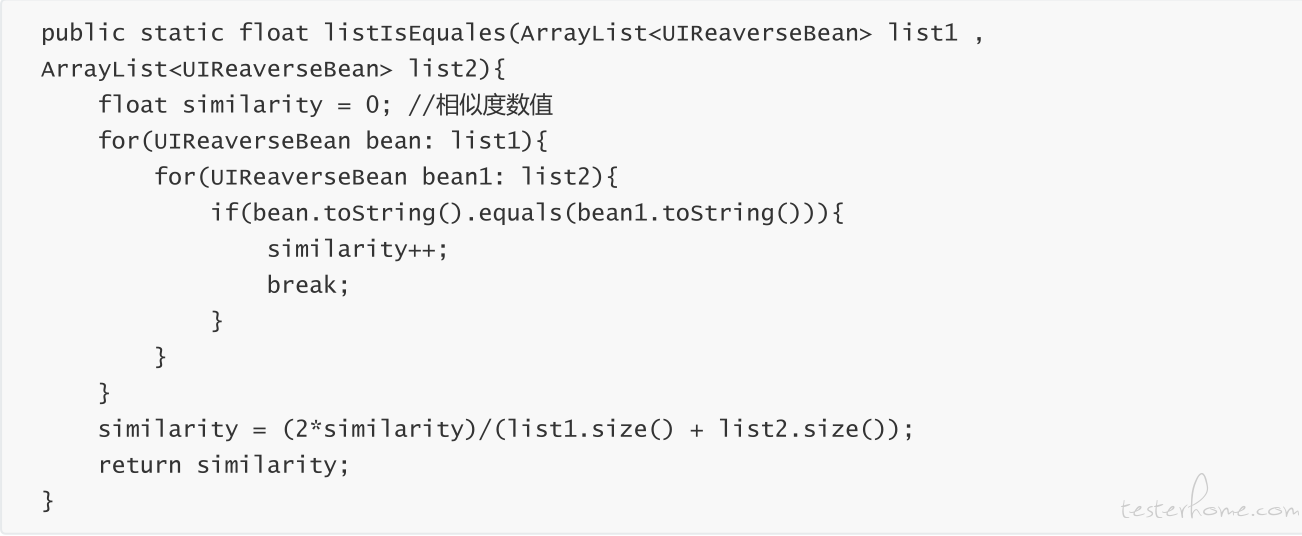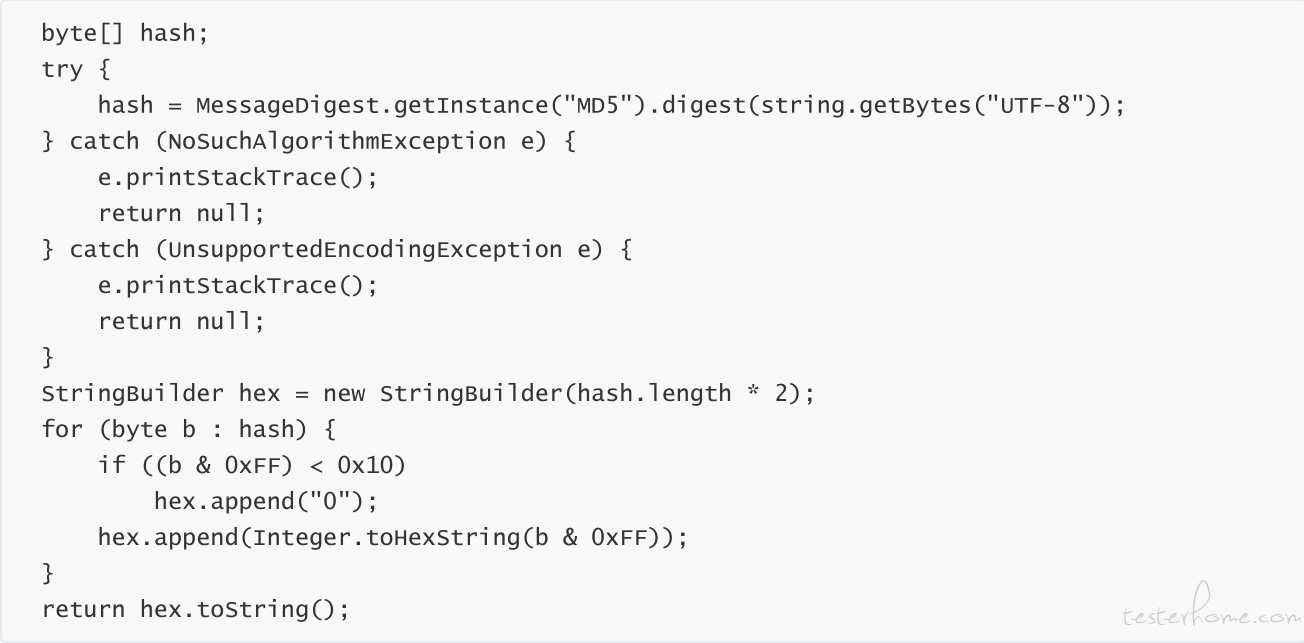## 实现步骤

1. 把图片缩小到 8*8尺寸，也就是总共64 像素2. 将当前的RGB图片转换为灰度图片，这个时候，图片只保留了图片的结构， 明暗等信息。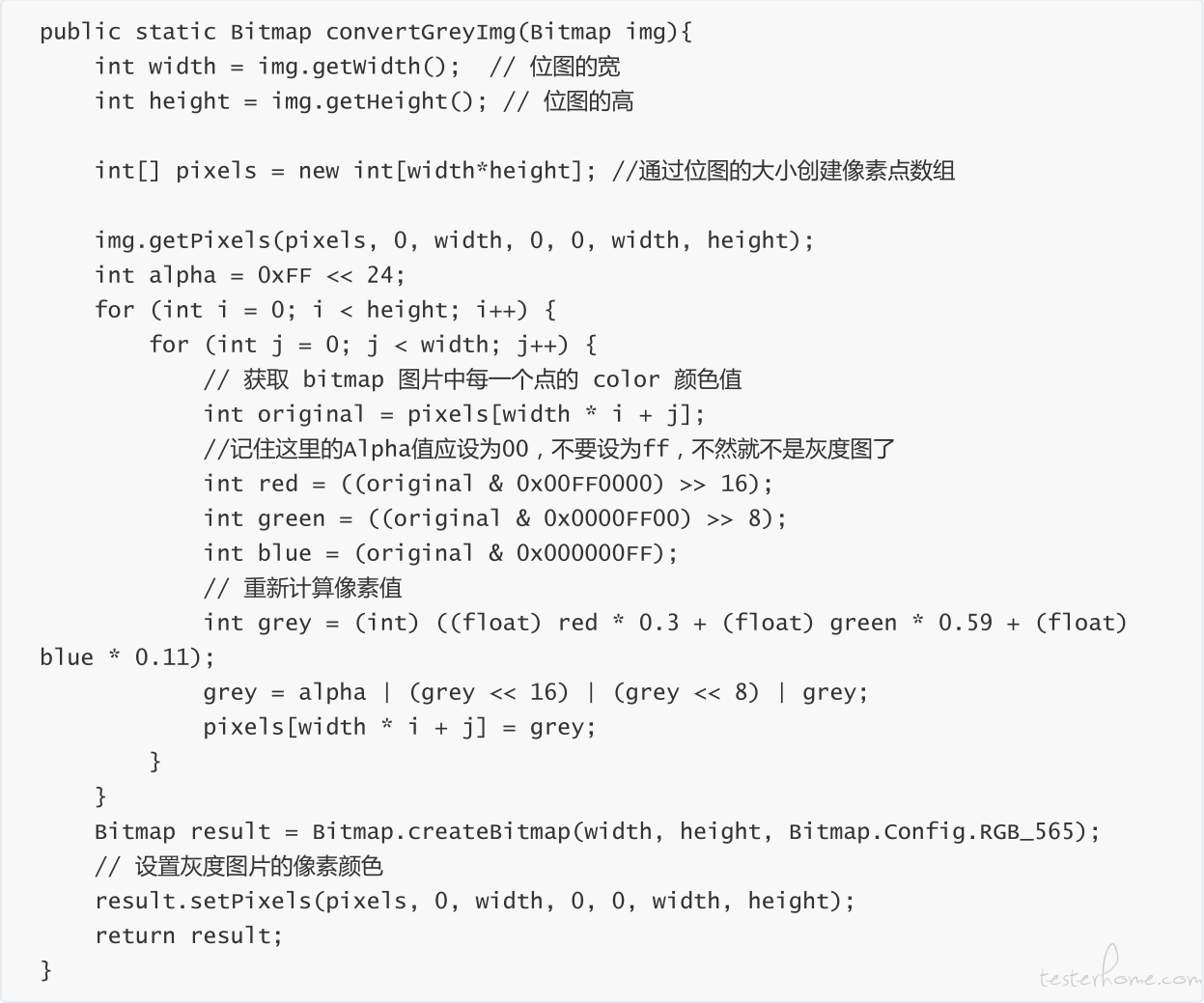1. 计算位图64个像素的平均灰度值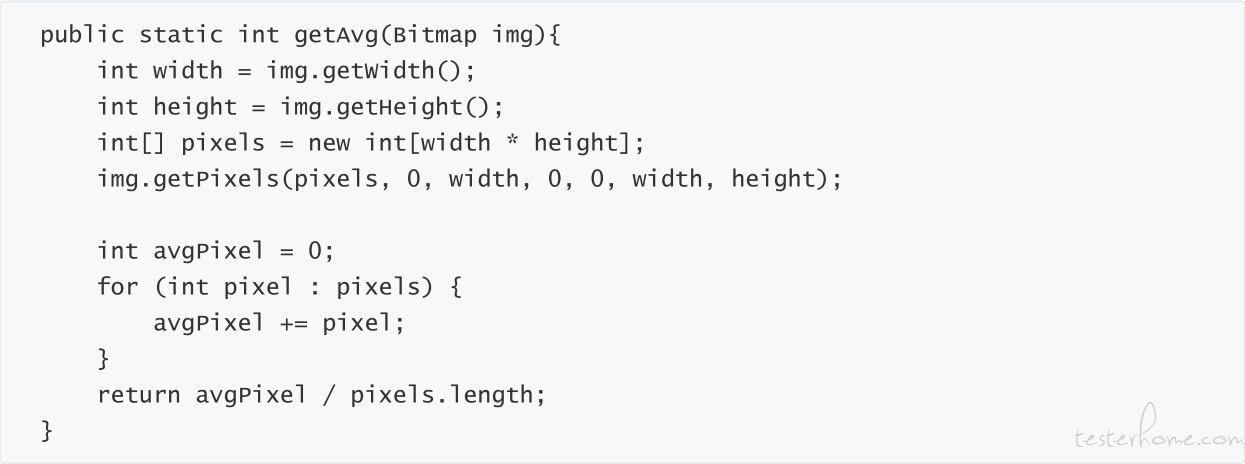2. 我们重新遍历位图，每个灰度值和平均灰度值进行比对， 大于等于平均灰度值为1， 小于平均灰度值为0，这样就会生成一个64位的数值。

3. 计算哈希值，这样生成的一个数值，就是每张图片的指纹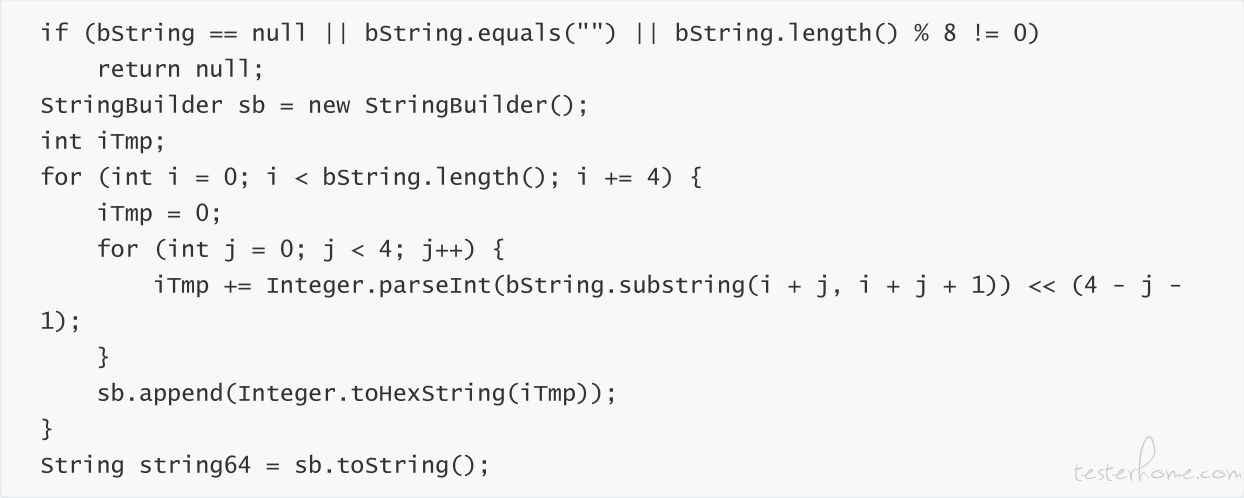4. 通过比对上面的指纹字符创，计算图片的相似度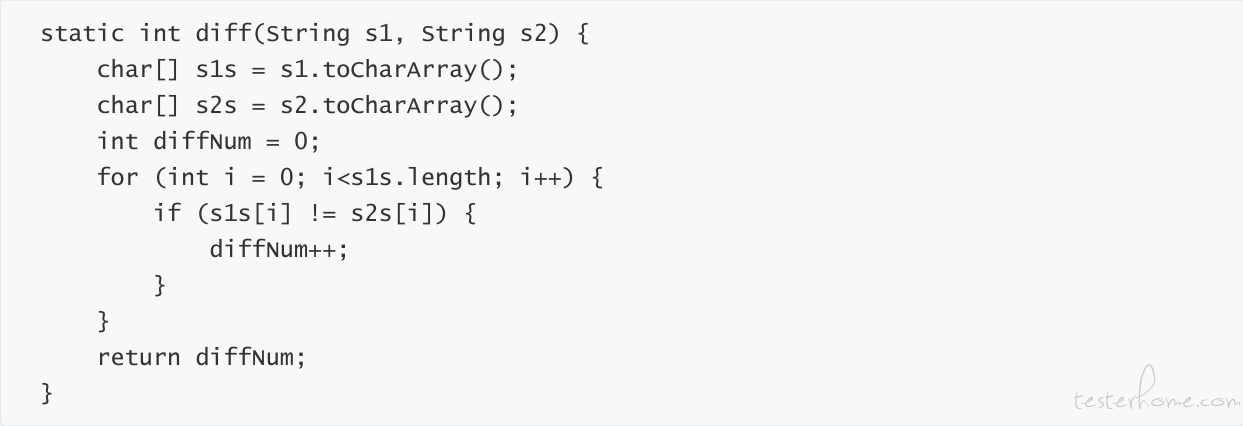diffNum 就是我们要获取的两张图片的相似度， diffNum< 5 图片很相似 diffNum > 10 图片完全不同。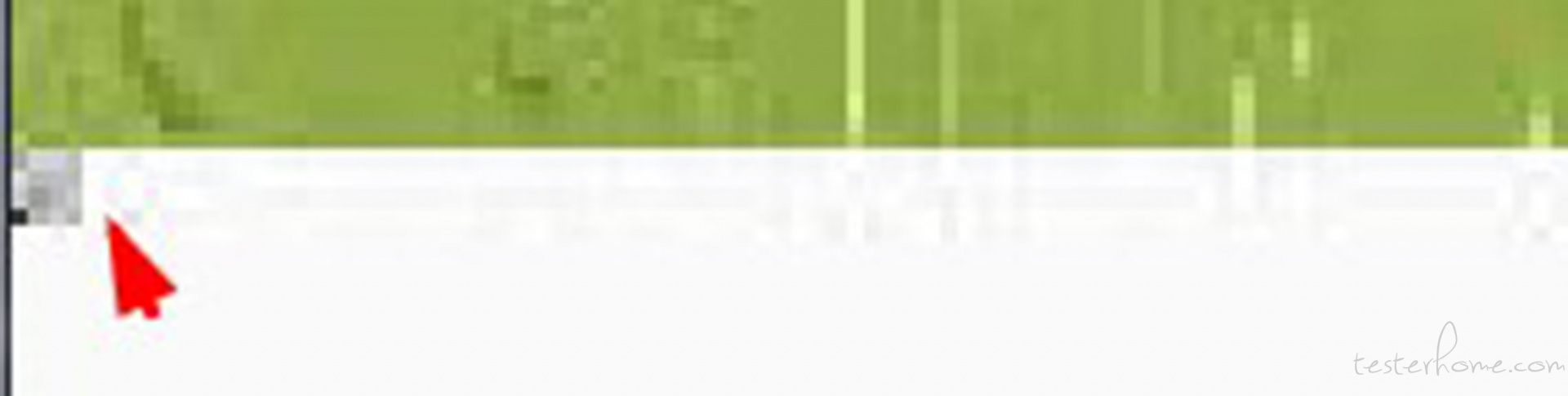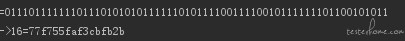``@Override    public boolean sameAs(IChimpImage other, double percent) {        BufferedImage otherImage = other.getBufferedImage();        BufferedImage myImage = getBufferedImage();        // Easy size check        if (otherImage.getWidth() != myImage.getWidth()) {            return false;        }        if (otherImage.getHeight() != myImage.getHeight()) {            return false;        }        int[] otherPixel = new int;        int[] myPixel = new int;        int width = myImage.getWidth();        int height = myImage.getHeight();        int numDiffPixels = 0;        // Now, go through pixel-by-pixel and check that the images are the same;        for (int y = 0; y < height; y++) {            for (int x = 0; x < width; x++) {                if (myImage.getRGB(x, y) != otherImage.getRGB(x, y)) {                    numDiffPixels++;                }            }        }        double numberPixels = (height * width);        double diffPercent = numDiffPixels / numberPixels;        return percent <= 1.0 - diffPercent;    }``

### 之前我写了个Python版本：

``def pic_diff(img_base, img_pre):    im_base = Image.open(img_base, 'r')      # print im.size    im_base = im_base.resize((8, 8), Image.ANTIALIAS).convert('L')     width = im_base.size      height = im_base.size     data_base = []      for h in range(0, height):          for w in range(0, width):              pixel = im_base.getpixel((w, h))             data_base.append(pixel)    im_pre = Image.open(img_pre, 'r')      # print im.size    im_pre = im_pre.resize((8, 8), Image.ANTIALIAS).convert('L')     width = im_pre.size      height = im_pre.size     data_pre = []      for h in range(0, height):          for w in range(0, width):              pixel = im_pre.getpixel((w, h))             data_pre.append(pixel)    count = 0    for x in xrange(0,64):        if data_base[x] == data_pre[x]:                count = count + 1    return count/(64.0)``